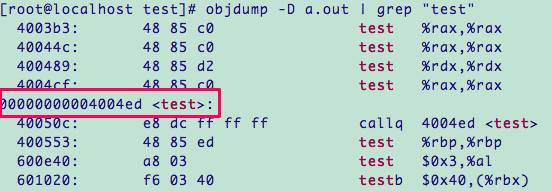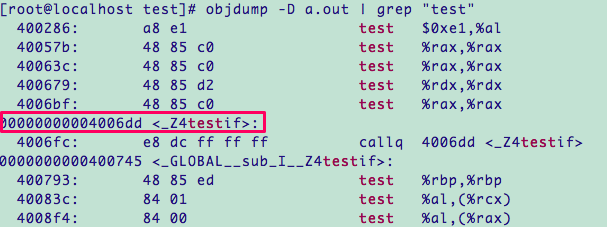# C++ | 函数重载

### 函数重载是什么

（其实写完这篇博客，觉得举这个例子不是很好..因为很多人并不是像我一样，先接触了 STL 再学习的 C++，看这篇博客的很多人应该并不理解 vector 之类的...然而已经写完了...）

（其实这里举这个例子可能会有些听不懂...虽然可以再讲很多别的例子..但是对我印象最深刻的函数重载就是这个= =）

1. 无参数；
2. 传一个 n 来设定 vector 的初始元素个数；
3. 传 n 设定元素个数，传 value 设定每个元素的初始值；
4. 是拷贝构造，传入一个 vector 的对象；
5. 传两个迭代器，设定第一个元素的地址与最后一个元素的下一位置的地址。


C++ 相对于 C 引入了函数重载，很方便的解决了我们很多问题，就像之前那个 vector 的几种构造方法一样，他们略微有一些差异，但最终实现的功能是一样的，我们可以根据他们的功能来记住这一类函数的名字，但若是每个函数都有不同的名字，调用时便会很不方便。

### 可以构成重载的要求

#### 参数列表的要求

1. 参数数目和类型
2. 参数的排列顺序


int test(int x);
double test(int x);


int test(int x);
double test(float x);


#### 1、类型需要完全匹配

	void test(int a);
void test(float f);


double t = 1;
test(t);


#### 2、注意引用

void test(int x);
void test(int& x);


    int a = 1;
test(a);


#### 3、const 指针类型匹配

void test(const char* s) {
cout << "test1" << endl;
}

void test(char* s) {
cout << "test2" << endl;
}

int main() {
const char a[] = "hello";
char b[] = "hello";
test(a);
test(b);
return 0;
}


test1
test2

void test(int x);
void test(const int x);


### 重载引用参数

void test(double & x);
void test(const double & x);
void test(double && x);


1. 形参为 可变的左值；
2. 形参为 可变的左值、不可变的左值 或 右值
3. 形参为 右值（最后这个书上说 rvalue，但翻译又说是左值，怀疑是写错了）。

void test(double& x) {
cout << "test1" << endl;
}

void test(const double& x) {
cout << "test2" << endl;
}

void test(double&& x) {
cout << "test3" << endl;
}

int main() {
double a = 1;
const double b = 2;
test(a);
test(b);
test(a + b);
return 0;
}


test1
test2
test3

void test(const double& x) {
cout << "test2" << endl;
}

int main() {
double a = 1;
const double b = 2;
test(a);
test(b);
test(a + b);
return 0;
}


test2
test2
test2

### 函数重载的原理

void test(int a, float b) {
// ...
}

int main() {
test(1, 2);
return 0;
}


linux 下执行以下语句来查看：

objdump -D a.out | grep "test"
a.out：是我生成的可执行文件名，如果不是这个名字，可修改下；
grep：由于汇编代码有点长..我就写了辣么几行代码就有好多啊..干脆就通过管道找起来方便点...

c的结果：c++的结果：